Updating search results...# 1 Cluster 5 Lessons

9 affiliated resources

# Search Resources

View
Selected filters: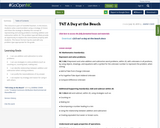Conditional Remix & Share Permitted
CC BY-NC-SA
Rating
0.0 stars

This resource is part of Tools4NCTeachers.

In this lesson, students solve a word problem using a strategy of choice and share the strategy to develop the concept of representing and solving problems involving addition and subtraction within 20. This problem type will likely provide an opportunity to explore the commutative property with students. This lesson format may be used with any problem type appropriate for the grade.

Subject:
Mathematics
Material Type:
Lesson
Lesson Plan
11/06/2019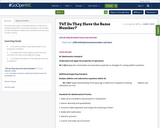Conditional Remix & Share Permitted
CC BY-NC-SA
Rating
0.0 stars

This resource is part of Tools4NCTeachers.

In this lesson, students explore the communitive property in the context of a word problem to determine if students have the same number of items

Subject:
Mathematics
Material Type:
Lesson
Lesson Plan
11/06/2019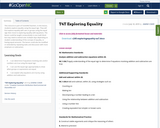Conditional Remix & Share Permitted
CC BY-NC-SA
Rating
0.0 stars

This resource is part of Tools4NCTeachers.

In this lesson, students explore equality using the equal sign. They will first explore equality with sets or groups using the equal sign then move to exploring equality with equations. The lesson could be taught consecutively in one math block but may need to extend over multiple days depending on student understanding of the concept of equality, or having equal amounts. The lesson could also be modified or extended by repeating tasks and discussion with more emphasis on subtraction.

Subject:
Mathematics
Material Type:
Lesson
Lesson Plan
11/06/2019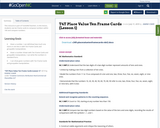Conditional Remix & Share Permitted
CC BY-NC-SA
Rating
0.0 stars

This resource is part of Tools4NCTeachers.

In this lesson, students use ten frame cards to compose numbers within 100 and compare numbers.

Subject:
Mathematics
Material Type:
Lesson
Lesson Plan
11/06/2019Conditional Remix & Share Permitted
CC BY-NC-SA
Rating
0.0 stars

This resource is part of Tools4NCTeachers.

In this lesson, students build fluency with recognizing and creating representations of numbers within 100. Students deepen their place value understanding as they work with ten frame cards. There are several variations built into the lesson for number ranges and response options.

Subject:
Mathematics
Material Type:
Activity/Lab
Lesson
Lesson Plan
11/06/2019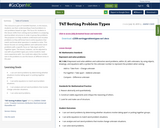Conditional Remix & Share Permitted
CC BY-NC-SA
Rating
0.0 stars

This resource is part of Tools4NCTeachers.

In this lesson, students use their understanding of word problems to sort problems based on type. The focus for students in this lesson shifts from solving word problems to analyzing word problem structures in order to group like problems. The purpose is to help students understand and visualize problems so they will know how to write equations that match and know what operation is needed to solve. This lesson focuses on sorting addition and subtraction word problems with a specific focus on Take Apart and Put Together types. The lesson, however, can be adjusted to use with any problem types you notice students need more experience with. You could also adjust the number size in the problems to use this lesson at different times in the year.

Subject:
Mathematics
Material Type:
Lesson
Lesson Plan
11/06/2019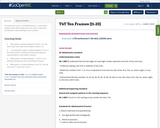Conditional Remix & Share Permitted
CC BY-NC-SA
Rating
0.0 stars

This resource is part of Tools4NCTeachers.

In this lesson, students work with double ten frame mats to represent and develop understanding of teen numbers.

Subject:
Mathematics
Material Type:
Activity/Lab
Lesson
Lesson Plan
11/06/2019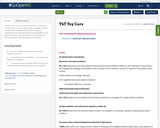Conditional Remix & Share Permitted
CC BY-NC-SA
Rating
0.0 stars

This resource is part of Tools4NCTeachers.

In this lesson, students solve a word problem using a strategy of choice and share the strategy to develop the concept of representing and solving problems involving addition and subtraction within 20. This lesson format may be used with any problem type appropriate for the grade.

Subject:
Mathematics
Material Type:
Lesson
Lesson Plan
11/06/2019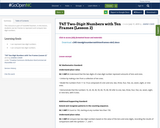Conditional Remix & Share Permitted
CC BY-NC-SA
Rating
0.0 stars

This resource is part of Tools4NCTeachers.

In this lesson, students use ten frames to represent and compare two-digit numbers.

Subject:
Mathematics
Material Type:
Lesson
Lesson Plan